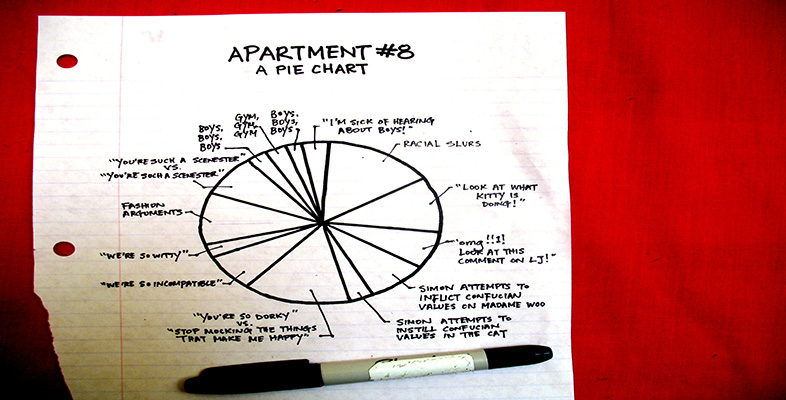More working with charts, graphs and tables

Start this free course now. Just create an account and sign in. Enrol and complete the course for a free statement of participation or digital badge if available.

Free course

# 8 Technical glossary

This glossary is intended to provide a basic explanation of how a number of common mathematical terms are used. Definitions can be very slippery and confusing and at worst can replace one difficult term with a large number of other puzzling concepts. Therefore, where an easy definition is available it is provided here, where this has not been possible an example is used. If you require more detailed or complete definitions, you should refer to one of the very good mathematical dictionaries that are available, or to one or more books listed in the bibliography.

Approximation: A numerical result given to a required level of accuracy, for example, to the nearest metre.

Average: The word ‘average’ is often used instead of the word ‘mean’ but it can have other interpretations so it is best to use the appropriate correct term; this is usually ‘mean’, but may be ‘median’ or ‘mode’ (see below).

Chart: Pictorial representation of data, e.g. pie chart, bar chart.

Continuous data: Values that cannot be given exactly. This data is usually a measurement like time, height or temperature.

Data: Items of numerical information, raw facts or statistics collected together for analysis.

Decimals: A way of expressing numbers using place value, e.g.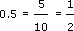,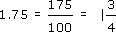Discrete data: Values that are clearly separated from one another, e.g. shoe sizes or modes of transport.

Fractions: Parts of a whole number, e.g.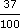,,, etc.

Graph: A drawing which depicts the relationship between data or values.

Inter-quartile range: The range of the values between the first and third quartile.

Mean: A typical value of a set of data, which is equal to the sum of the values in the set divided by the number of values in the set.

Median: The middle point of a set of values arranged in ascending or descending order.

Mode: The most common value in a set of data.

Percentage: A proportion of a hundred parts, e.g.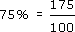Quartile: Each of the three values that divide a set of data into four equal groups.

Ratio: A comparison of one quantity with another.

Scale: A series of marks at regular intervals on a line used for measuring, e.g. the divisions on the axis of a graph or the degree markings on a thermometer.

Value: A numerical amount.

LDT_4